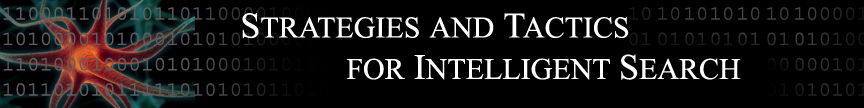ALGORITHMS - BRANCH AND BOUND

The branch and bound algorithm is a pruning technique that allows us to examine a given path, decide whether to keep considering it or to disregard it based on the evaluation of the path in comparison with others so far. As mentioned above, the branch and bound search algorithm considers the best path so far, while other algorithms look at the best successor step in a given path. The path cost for each route explored is stored and used to sort the choices.

The branch and bound is a depth first search based algorithm that considers the best path so far, backtracking whenever it encounters a node whose value is larger than its current upper bound. The algorithm terminates when all paths have been explored or pruned. The algorithm is both optimal and complete, as in it will search all the possible solutions and find the best one. The advantages of the search are low memory use and the availability of the best partial path so far.

Fig. 1 (demo from http://www.utia.cas.cz/user_data/PR_dept/fs/fs_algorithms.html#References)
Our task is to select 2 items out of the total number of 6 items (as shown in the figure above) so as to
maximize an adopted criterion function. During demonstrations the red color denotes criterion value
computation, yellow color denotes a much faster criterion value prediction.

In short, branch and bound is an algorithm technique to find the optimal solution by keeping the best solution found so far. If a partial solution examined at a given point cannot do better than the best, work on it is abandoned.